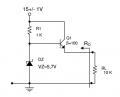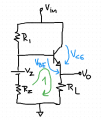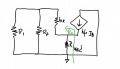# Voltage Regulators - Problem 4.b

#### PsySc0rpi0n

Joined Mar 4, 2014
1,702
Ok, my next problem is related with the previous one!

The new circuit is an improved version of the previous one!

I think this is supposed to demonstrate us that this new circuit has a lot better voltage regulation compared to the previous circuit!To find Vo, which is the voltage drop across RLoad, I'm firstly replacing the zener by a voltage source in series with a resistor (100 Ω).Then I tried to write an equation for that green closed loop:

Vbe + Ie*RLoad = Vz + Iz*Rz

But I have not enough data to find Ve = Vo.

Then I tried to write another equation for the right side of the circuit starting in Vin all way down to GND:

But I still have not enough data! I still have 3 unknowns and only 2 equations! And the transistor currents relations for the active zone are not taking me anywhere too, I guess!

A small push would be appreciated!

#### Jony130

Joined Feb 17, 2009
5,445
The hard way

Vcc = I1*R1 + I2*Rz+Vz
(1)

I1 = I2+Ib (2)

(3)

And Ib = Ie/(β+1) (4)

Or use a Thevenin theorem and find the voltage at the transistor base without transistor in the circuit (Vth and Rth).

•anhnha

#### PsySc0rpi0n

Joined Mar 4, 2014
1,702
I thought about those 3 equations of yours, 1, 2 and 4. And what about that other one I wrote? Is it correct? Could I use it anywhere else where it would be needed? I mean, the last problem, I end up writing equations that could not be written due to the nodes and branches stuff!

What about that equation of mine? Could it be written if it was needed?

Last edited:

#### Jony130

Joined Feb 17, 2009
5,445
What about that equation of mine? Could it be written if it was needed?
In this case (you have a transistor that work as a voltage follower) this equation involving Vce is useless, because the collector-emitter act here as a current source. But this is only my opinion.

#### PsySc0rpi0n

Joined Mar 4, 2014
1,702
Ok, I already did the math for the 3 values of Vin, 14 V, 15 V and 16V...

I got Vo1 = 5.78V, Vo2 = 5.87 V and Vo3 = 5.96 V by means of Ie*RLoad!

I can see that the variation is small! I need to compare it to the variation of the previous circuit to check that in fact this circuit has better regulation!

Now, I'm going to try to go for Ro using the bjt hybrid model!

#### Jony130

Joined Feb 17, 2009
5,445
Now, I'm going to try to go for Ro using the bjt hybrid model!
That should be fairly easy job if you know Ro for a common collector amplifier (emitter follower).

Last edited:
•anhnha

#### PsySc0rpi0n

Joined Mar 4, 2014
1,702
I just got home... Going to try the Ro calculation!

#### PsySc0rpi0n

Joined Mar 4, 2014
1,702
@Jony130 , shouldn't this Vo be more stable than the one from the previous circuit?

Also, for the 1st circuit I have calculated each Vo for each Vin of 14 V, 15 V and 16 V and I got the following results:

14 V -> Vo = 5.766 V
15 V -> Vo = 5.855 V
16 V -> Vo = 5.946 V

For this circuit I did the same and got this:

14 V -> Vo = 5.87 V
15 V -> Vo = 5.78 V
16 V -> Vo = 5.91 V

I was expecting that the Vo of this circuit oscillates less than the previous one, but I'm not observing that! Also, Vo for Vin = 15 V is lower than the Vo for Vin = 14 V. This didn't happened with the previous circuit where the next Vo was higher than the previous one!

#### Jony130

Joined Feb 17, 2009
5,445
And Vz is 5V as before?? and Vbe is ??

#### PsySc0rpi0n

Joined Mar 4, 2014
1,702
And Vz is 5V as before?? and Vbe is ??
For the 1st circuit Vz = 5 V, the 2nd circuit Vz = 5.7 V and I used Vbe = 0.67 V for both circuits!

#### Jony130

Joined Feb 17, 2009
5,445
Ok I see, but adding a BJT do not improve line regulation (ΔVout/ΔVsup). Because the BJT is just a voltage follower and the main cause of Vo instability is a Zener diode (large Rd and change in Iz current due to Vsup changes ).

#### PsySc0rpi0n

Joined Mar 4, 2014
1,702
Ok I see, but adding a BJT do not improve line regulation (ΔVout/ΔVsup). Because the BJT is just a voltage follower and the main cause of Vo instability is a Zener diode (large Rd and change in Iz current due to Vsup changes ).

Hum, I see, but I was not expecting "instability" in linear mathematical equations, that's what I find strange! Anyway, I thought that these 2 circuits were to show us that the second would provide much better voltage regulation than the first one, no?

#### Jony130

Joined Feb 17, 2009
5,445
Hum, I see, but I was not expecting "instability" in linear mathematical equations, that's what I find strange!
But you made a small error
14 V -> Vo = 5.78 V
15 V -> Vo = 5.87 V
16 V -> Vo = 5.96 V
I thought that these 2 circuits were to show us that the second would provide much better voltage regulation than the first one, no?
No, the main advantage is that now the circuit can provide a large current to the load. Because now the BJT provides a current gain.
Therefore ILoad = Ie = Ib + Ib*β = (β+1)*Ib
So now the change in the load current will cause (β+1) smaller change in the zener current (Iz).
And this allows us to increase the max load current that we can drawn from the circuit.

Last edited:

#### PsySc0rpi0n

Joined Mar 4, 2014
1,702
But you made a small error
14 V -> Vo = 5.78 V
15 V -> Vo = 5.87 V
16 V -> Vo = 5.96 V

No, the main advantage is that now the circuit can provide a large current to the load. Because now the BJT provides a current gain.
Therefore ILoad = Ie = Ib + Ic*β = (β+1)*Ib
So now the change in the load current will cause (β+1) smaller change in the zener current (Iz).
And this allows us to increase the max load current that we can drawn from the circuit.
Yeah, I made the calcs firstly for 15 V, then I thought the 5.87 V was for 14 V.

You mean ILoad = Ie = Ib + Ib*β = (β+1)*Ib, right? Ok... I see the point!

Ok, I have drew a schematic for the equivalent model to try to find Ro. I got this:#### Jony130

Joined Feb 17, 2009
5,445
You mean ILoad = Ie = Ib + Ib*β = (β+1)*Ib, right? Ok... I see the point!
Yep, this is exactly what I mean.

Ok, I have drew a schematic for the equivalent model to try to find Ro. I got this:
Remove the load resistor and you will be fine.
Also without any calculation you can look into AOE and find out that ro = Rs/(β+1) + re or (Rs + hie)/(β + 1), where Rs = R1||Rz.

#### PsySc0rpi0n

Joined Mar 4, 2014
1,702
I'm not getting anywhere with this!

I understand that everything that is "behind" the emitter needs to be divided by (β+1) because of the emitter effect, but I still need to get it stpe-by-step to understand how we get the result!

#### Jony130

Joined Feb 17, 2009
5,445
Well, ok here you have

Rs = R1||Rz

Ib + Ib*β = -Ix

Ib*(β + 1) = -Ix

Ib = -Vx/(Rs + hie)

-Ix = -Vx/(Rs + hie) * (β + 1)

Multiply both sides by -1

Ix = Vx/(Rs + hie)*(β + 1)

And if Vx = 1 V---- > ro = 1/Ix = (hie + Rs)/(β + 1)

Last edited:
•PsySc0rpi0n

#### PsySc0rpi0n

Joined Mar 4, 2014
1,702
Well, ok here you have

Rs = R1||Rz

Ib + Ib*β = -Ix

Ib*(β + 1) = -Ix

Ib = -Vx/(Rs + hie)

-Ix = -Vx/(Rs + hie) * (β + 1)

Multiply both sides by -1

Ix = Vx/(Rs + hie)*(β + 1)

And if Vx = 1 V---- > ro = 1/Ix = (hie + Rs)/(β + 1)
I just have a question about Ib.
As for the equivalent circuit, and as far as I see, the current flowing through hie is Ib but is not the same Ib that we had before the equivalent circuit, right?

I'm not sure if I'm understanding this correctly but by your equations looks like Ib = IR1 + IRz, no ?

#### Jony130

Joined Feb 17, 2009
5,445
I'm not sure if I'm understanding this correctly but by your equations looks like Ib = IR1 + IRz, no ?
No, I find a equivalent resistance Rs = R1||Rz. And this equivalent resistance is connected in series with hie. If so, this mean that Ib flow through Rs. Because in a series circuit the current is the same for all of elements.
But you can always use brutal force = nodal analysis.

Last edited: